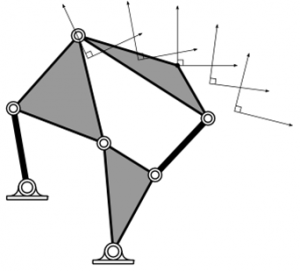## Finite Root GenerationFinite Root Generation (FRG) is a method to collect all roots of a polynomial system.  Particularly, FRG is useful for solving large systems of equations associated with mechanism synthesis problems.  It uses homotopy continuation to accomplish this goal. Homotopy continuation finds all roots (endpoints) of a target system by first constructing a start system from […]

## Homotopy and Mechanism DesignPlanar, spherical, and spatial linkage design requires the formulation of synthesis equations. These equations are often polynomials or can be converted to polynomials. The degree of these polynomial systems increases rapidly with mechanism complexity. For example, the synthesis equations of a planar RR constraint used to construct a four-bar linkage that reaches its maximum of […]

## Synthesis of Six-barsThe six-bar linkage is the next simplest 1-DOF planar mechanism after the four-bar. However, designing these mechanisms presents a challenging set of synthesis equations. For example, a four-bar motion generator is determined by a polynomial system that has 4 isolated roots (of which one is the origin), where each root represents a potential design. On […]